# Bayesian Data Analysis with brms

In this post, we’ll walk through the Bayesian workflow for data analysis using the R package brms. To get started, we need to install Stan and brms. This involves a couple steps. Here are the instructions for MacOS and Windows which you can also find here (https://learnb4ss.github.io/learnB4SS/articles/install-brms.html):

Note: this installation take about 20 minutes to complete.

## MacOS Installation Instructions

To install brms on MacOS, you have to do four steps:

1. Make sure you are running the latest version of R (4.0 or later)
2. Install rtools
3. Install Rstan
4. Install brms

### 2. Install rtools

In order to install rtools on MacOS, we have to install the Xcode Command Line Tools and gfortran.

First, let’s install the Xcode Command Line Tools. Open Terminal (Finder > Applications > Terminal), then type in the following command and press enter:

xcode-select --install

After this finishes (it might take a while), we can install gfortran. If you have an Intel Mac download and install this file: https://github.com/fxcoudert/gfortran-for-macOS/releases/tag/8.2. If you have a Mac with an Apple chip, download and install this file: https://github.com/fxcoudert/gfortran-for-macOS/releases/tag/11-arm-alpha2.

### 3. Install Rstan

install.packages("rstan", repos = "https://cloud.r-project.org/", dependencies = TRUE)

Wait! Did you install it right? Run this to check:

example(stan_model, package = "rstan", run.dontrun = TRUE)

### 4. Install brms

install.packages("brms")

## Windows Installation Instructions

### 2. Install rtools

To install rtools, go here, download the file (.exe) and install it: https://cran.r-project.org/bin/windows/Rtools/

### 3. Install Rstan

install.packages("rstan", repos = "https://cloud.r-project.org/", dependencies = TRUE)

Wait! Did you install it right? Run this to check:

example(stan_model, package = "rstan", run.dontrun = TRUE)

### 4. Install brms

install.packages("brms")

# Preparation

To get started we’ll need to load the tidyverse and brms R packages and load in the data.

Our goal is to estimate the relationship between engine size and highway MPG.

library(tidyverse)
## ── Attaching packages ─────────────────────────────────────── tidyverse 1.3.1 ──
## ✓ ggplot2 3.3.5     ✓ purrr   0.3.4
## ✓ tibble  3.1.6     ✓ dplyr   1.0.7
## ✓ tidyr   1.1.4     ✓ stringr 1.4.0
## ✓ readr   2.1.1     ✓ forcats 0.5.1
## ── Conflicts ────────────────────────────────────────── tidyverse_conflicts() ──
## x dplyr::lag()    masks stats::lag()
library(brms)
## Loading required package: Rcpp
## Loading 'brms' package (version 2.16.3). Useful instructions
## can be found by typing help('brms'). A more detailed introduction
## to the package is available through vignette('brms_overview').
##
## Attaching package: 'brms'
## The following object is masked from 'package:stats':
##
##     ar
data("mpg")

summary(mpg)
##  manufacturer          model               displ            year
##  Length:234         Length:234         Min.   :1.600   Min.   :1999
##  Class :character   Class :character   1st Qu.:2.400   1st Qu.:1999
##  Mode  :character   Mode  :character   Median :3.300   Median :2004
##                                        Mean   :3.472   Mean   :2004
##                                        3rd Qu.:4.600   3rd Qu.:2008
##                                        Max.   :7.000   Max.   :2008
##       cyl           trans               drv                 cty
##  Min.   :4.000   Length:234         Length:234         Min.   : 9.00
##  1st Qu.:4.000   Class :character   Class :character   1st Qu.:14.00
##  Median :6.000   Mode  :character   Mode  :character   Median :17.00
##  Mean   :5.889                                         Mean   :16.86
##  3rd Qu.:8.000                                         3rd Qu.:19.00
##  Max.   :8.000                                         Max.   :35.00
##       hwy             fl               class
##  Min.   :12.00   Length:234         Length:234
##  1st Qu.:18.00   Class :character   Class :character
##  Median :24.00   Mode  :character   Mode  :character
##  Mean   :23.44
##  3rd Qu.:27.00
##  Max.   :44.00

# Picking Priors

Plotting priors is the best way to understand how influential they are (this is especially true when we are using link functions like Logit and log). There are two ways to do this. First we can simply plot the prior densities. Second, we can preform full prior predictive simulation. Let’s try both.

Remember, the goal here is to pick a prior for the effect of engine size on a vehicle’s highway MPG. Engine size is measured in liters so what sounds reasonable? Would an increase in the size of an engine by 1 liter decrease MPG by 1000? Probably not. Maybe more like 1-3 MPG?

## Plotting Prior Densities

# Some MPG Data
x <- seq(from = 0, to = 50, by = 0.1)

# Frequentist Priors
curve(dunif(x, min = -1000000, max = 1000000), from = -1000000, to = 1000000)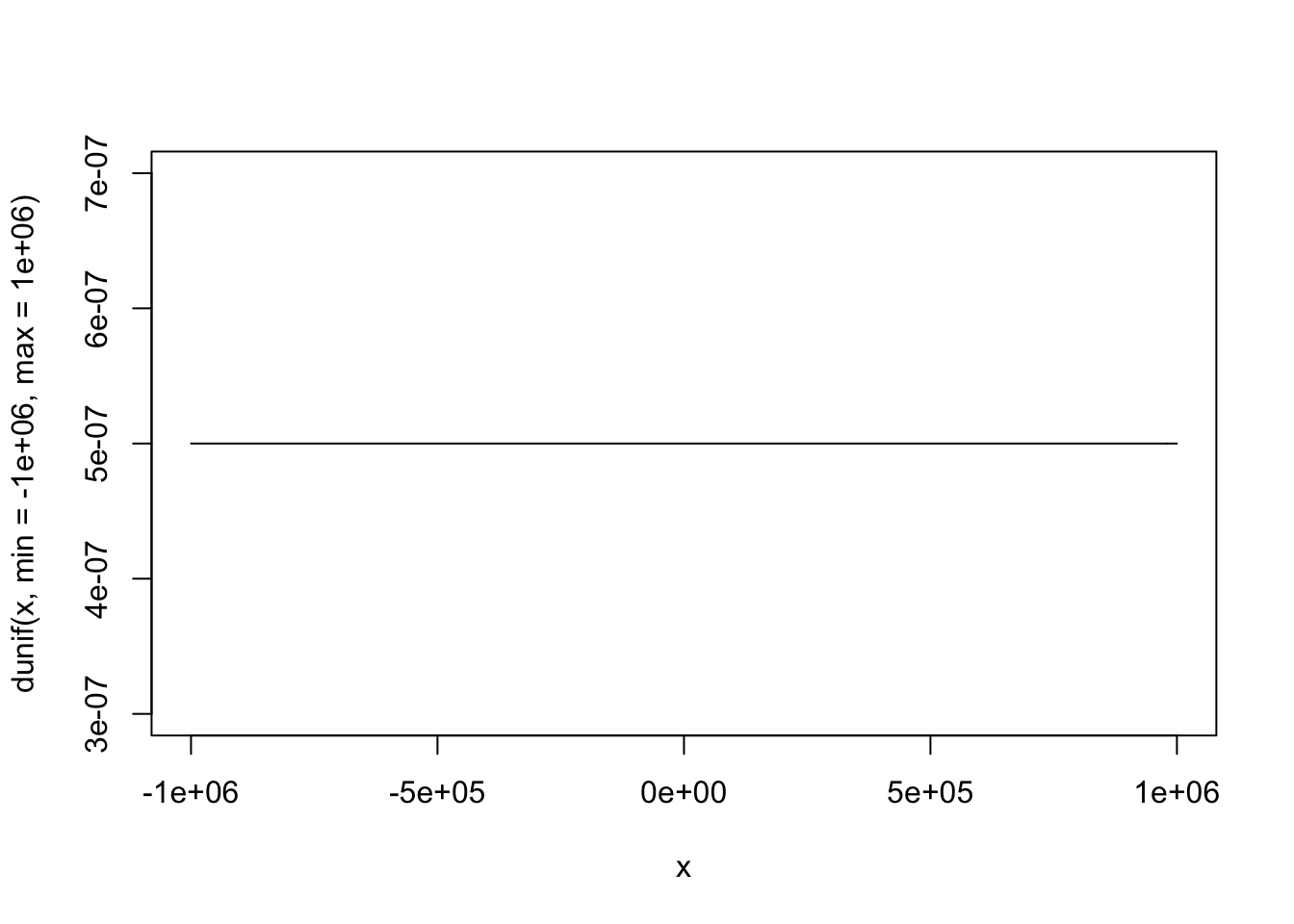# Flat Priors
curve(dunif(x, min = -100, max = 100), from = -110, to = 110)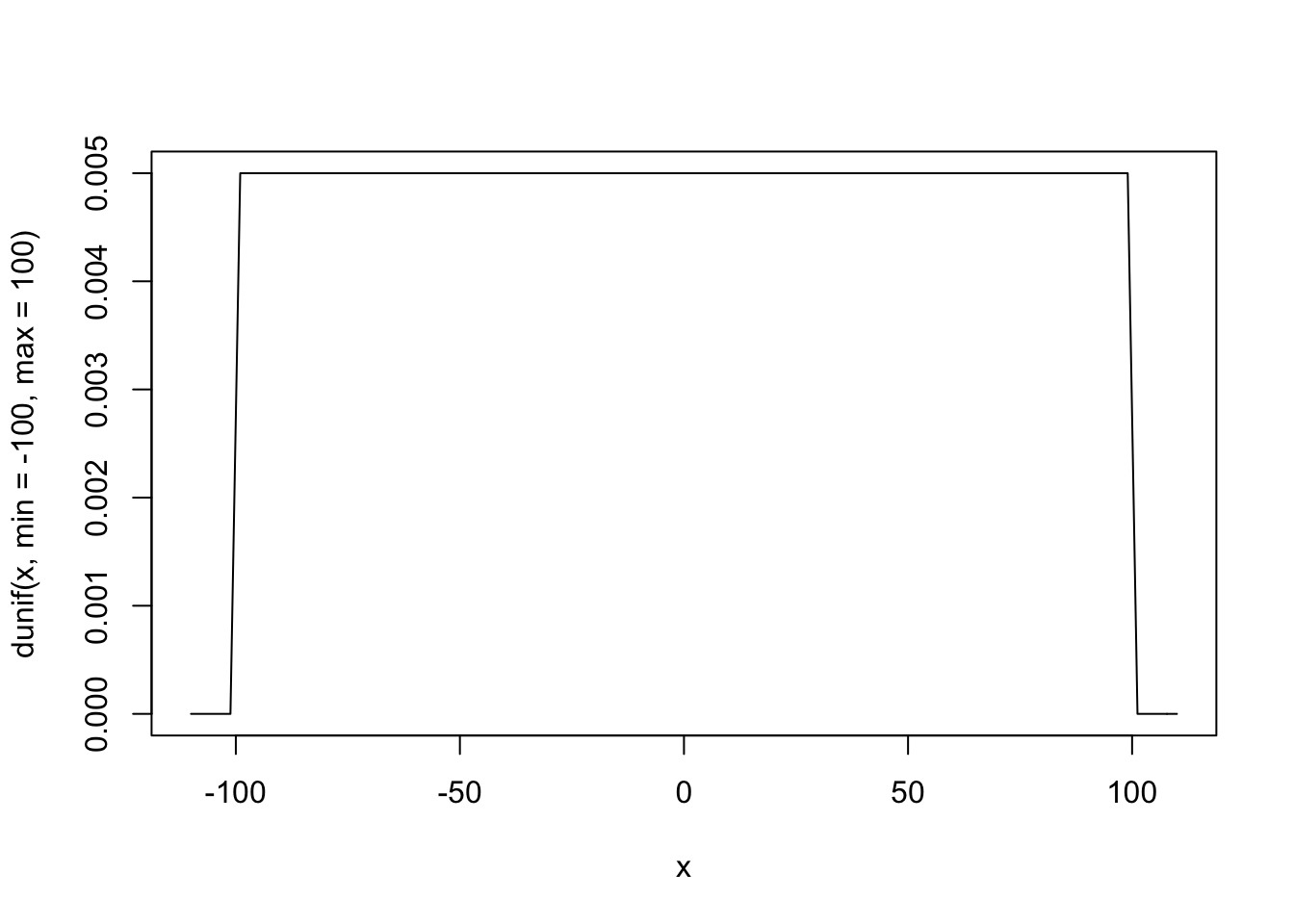# Weakly Informative Priors
curve(dnorm(x, mean = -5, sd = 20), from = -50, to = 50)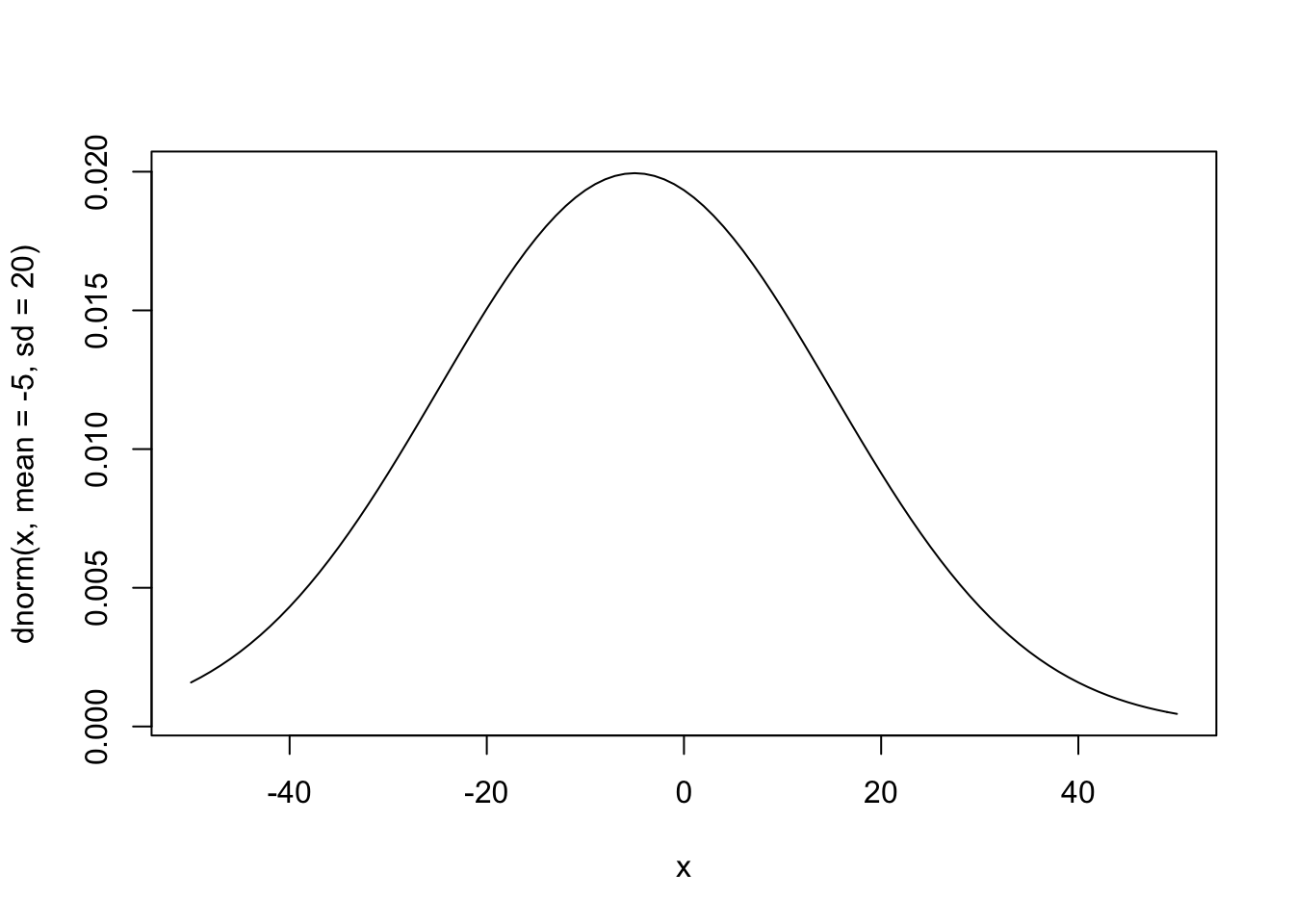# Informative Priors
curve(dnorm(x, mean = -3, sd = 2), from = -10, to = 10)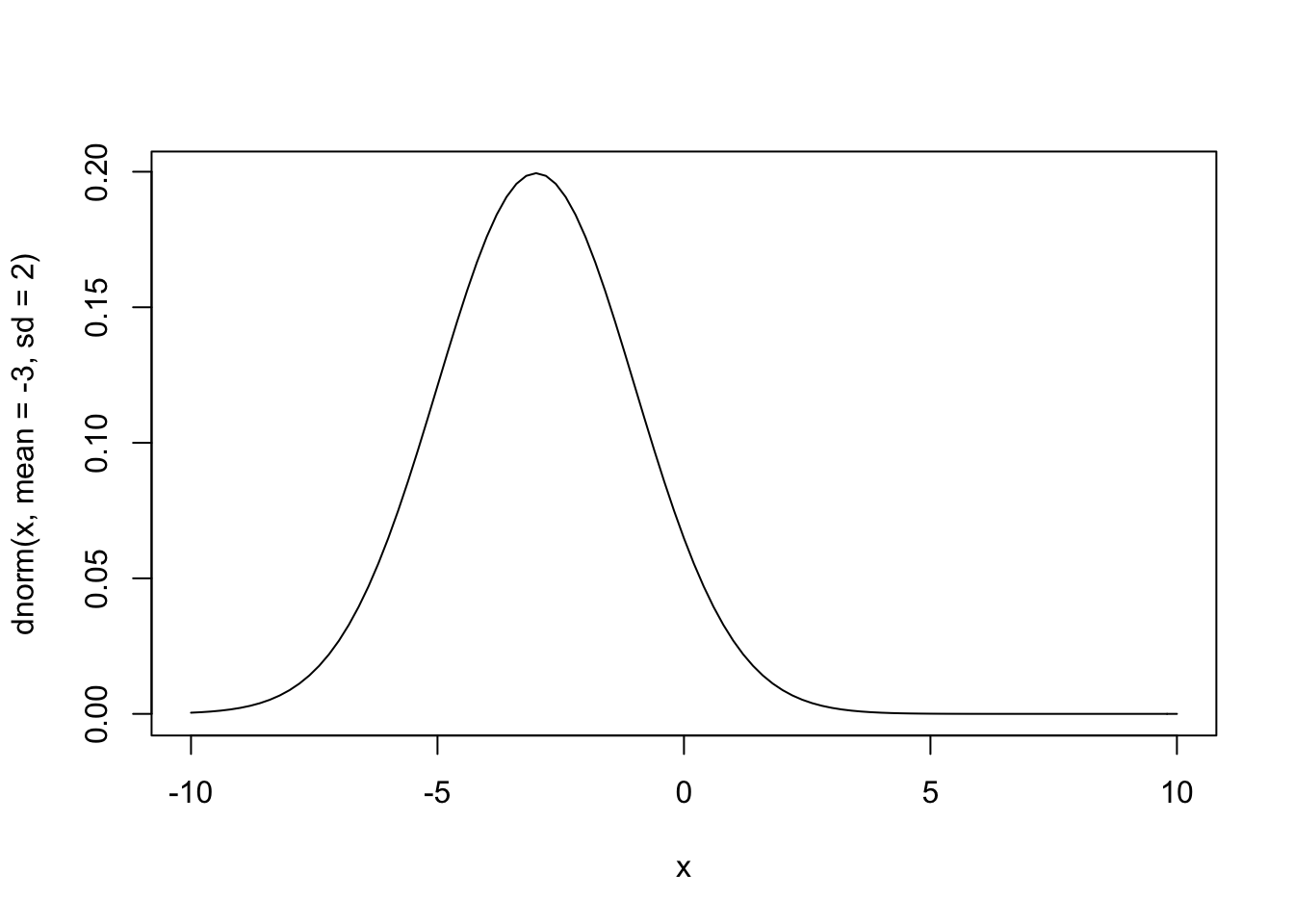## Prior Predictive Simulations

Another option for picking priors is to build a complete prior predictive simulation. Basically, this means that we run the model we are interested in without the data. To do this, we also need to pick a prior for the intercept.

To see how priors affect what the model knows we’ll look at “frequentist” priors, weakly informative priors, and very informative priors.

It’s easy to pick a prior for the intercept if we remember that the intercept is just the mean of the outcome variable. What do you think the average highway MPG is for cars in the US? 1000, -1000, 15, 30? Remember that frequentist analyses use completely uninformative priors - all values are possible.

### “Frequentist” Priors

Frequentist models assume that all values are equally likely. In other words, they give no information to the model to improve prediction.

# Number of simulations
sample.size <- 100

# Prior for the intercept
intercept <- runif(sample.size, min = -10000000, max = 10000000)

# Prior for the effect of engine size
b_1 <- runif(sample.size, min = -10000000, max = 10000000)

# Variables
x <- mpg$displ xbar <- mean(mpg$displ)

# Prior Predictive Simulation
plot(NULL, xlim = range(mpg$displ), ylim = c(-100, 200), xlab = "Engine Size", ylab = "Highway MPG") abline(h = 0, lty = 1, lwd = 1.5, col = "black") abline(h = 142, lty = 1, lwd = 1.5, col = "black") for (i in 1:sample.size) { curve(intercept[i] + b_1[i] * (x - xbar), from = min(mpg$displ),
to = max(mpg$displ), add = TRUE, col = "gray") }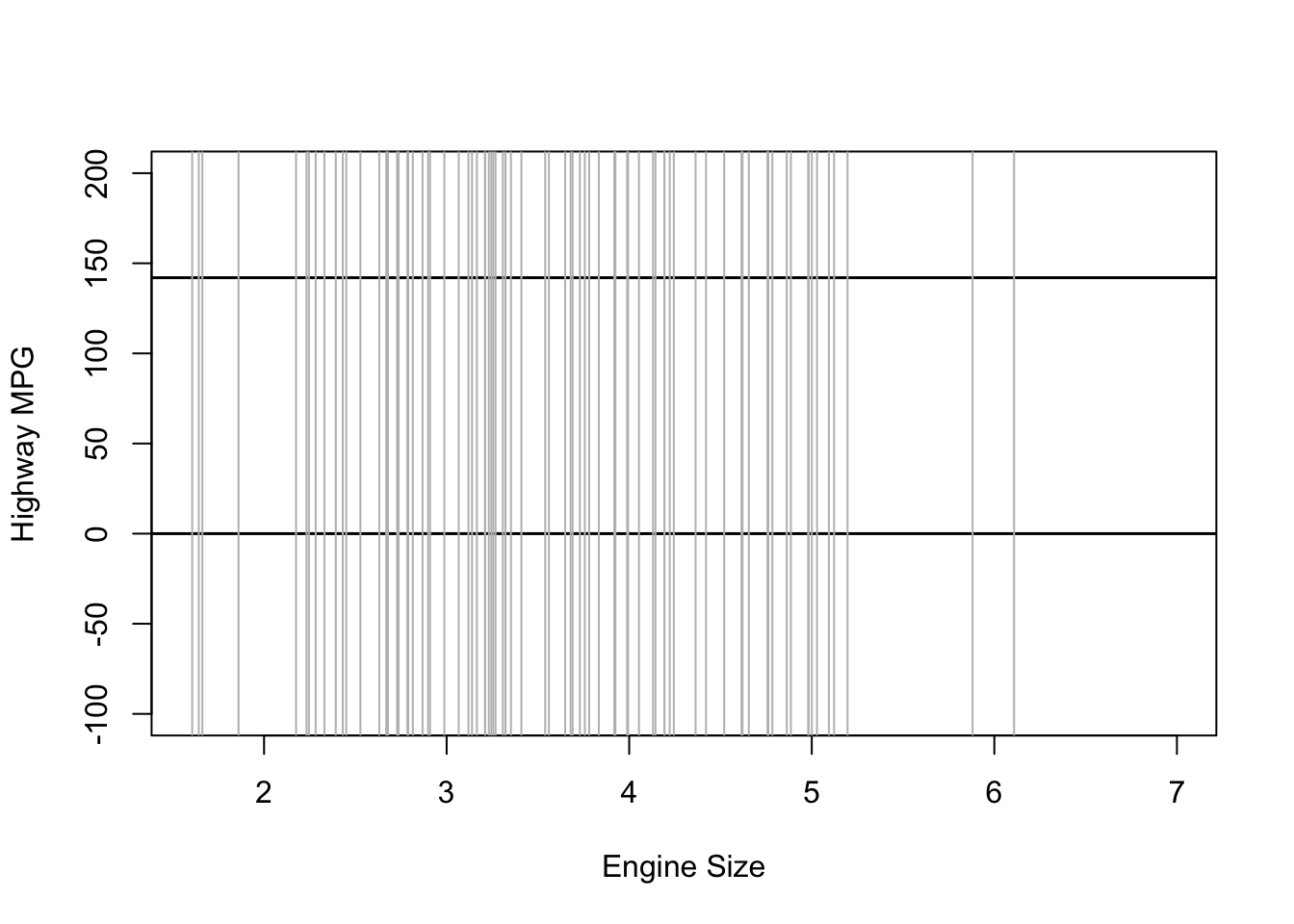### Weakly Informative Priors Using weakly informative priors is almost always a better option. In nearly every case, we at least know something about the kind of effects/estimates we expect to find. In the example of engine size and MPG, an increase of one liter in engine size probably won’t affect highway MPG but 1,000 or even 100. My guess is that the average car MPG is probably around 20, so we’ll use that as the mean for the prior on our intercept. Since I’m not too certain in this guess, I’ll still use a big standard deviation. As for the effect of engine size, I expect the effect to be negative and not too large so I’ll use -5 with a wide standard deviation. # Number of simulations sample.size <- 100 # Prior for the intercept intercept <- rnorm(sample.size, mean = 20, sd = 20) # Prior for the effect of engine size b_1 <- rnorm(sample.size, mean = -5, sd = 20) # Variables x <- mpg$displ
xbar <- mean(mpg$displ) # Prior Predictive Simulation plot(NULL, xlim = range(mpg$displ), ylim = c(-100, 200),
xlab = "Engine Size", ylab = "Highway MPG")
abline(h = 0, lty = 1, lwd = 1.5, col = "black")
abline(h = 142, lty = 1, lwd = 1.5, col = "black")
for (i in 1:sample.size) {
curve(intercept[i] + b_1[i] * (x - xbar), from = min(mpg$displ), to = max(mpg$displ), add = TRUE, col = "gray")
}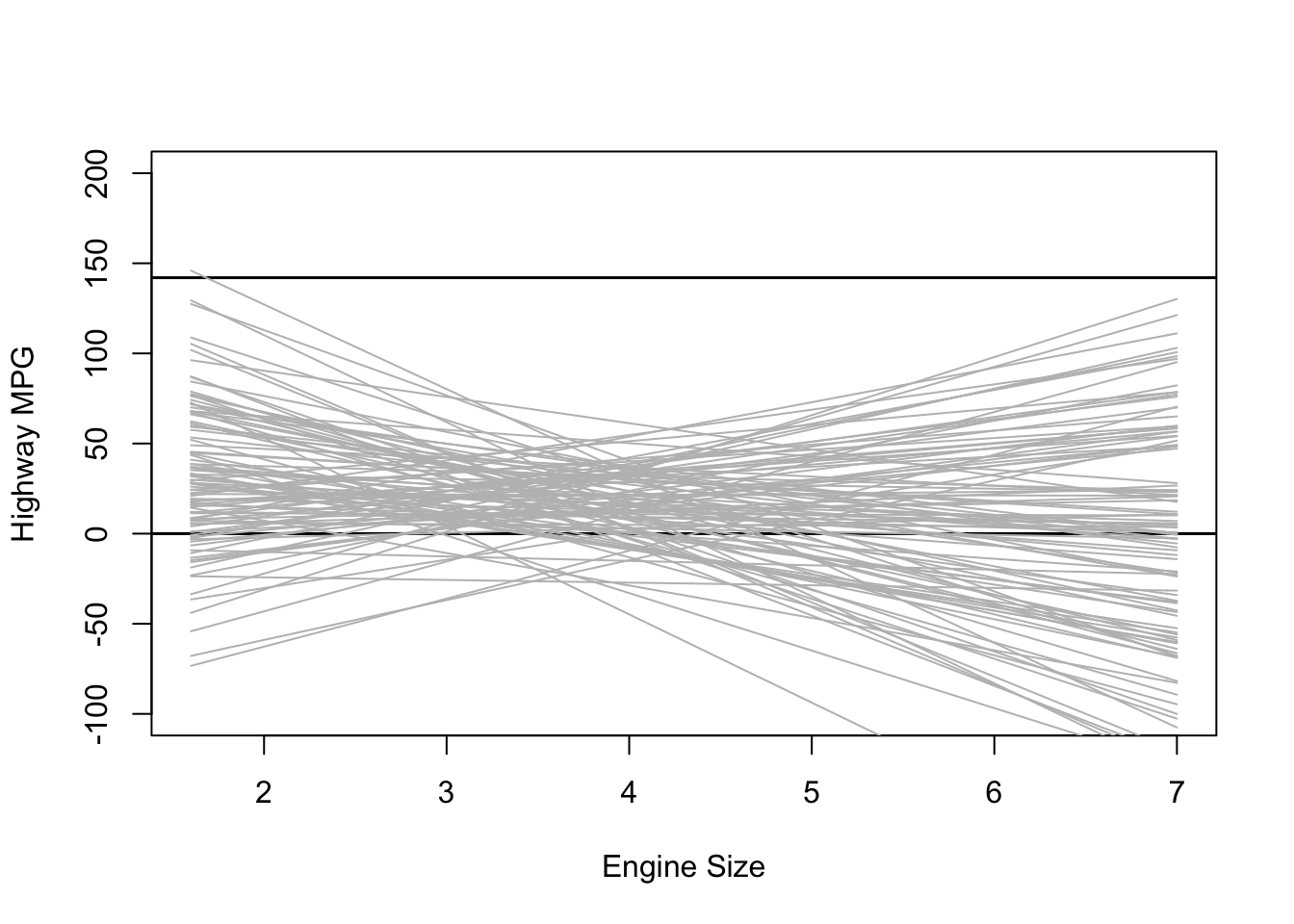This plot shows the predicted highway MPG based only on our choice of priors for the intercept and slope. It’s not too bad, but there are definitely some outliers.

### Informative Priors

What happens when we use really informative priors? Here is some data on the average MPG for different cars:# Prior for the intercept
intercept <- rnorm(sample.size, mean = 25, sd = 5)

# Prior for the effect of engine size
b_1 <- rnorm(sample.size, mean = -3, sd = 2)

# Variables
x <- mpg$displ xbar <- mean(mpg$displ)

# Prior Predictive Simulation
plot(NULL, xlim = range(mpg$displ), ylim = c(-100, 200), xlab = "Engine Size", ylab = "Highway MPG") abline(h = 0, lty = 1, lwd = 1.5, col = "black") abline(h = 142, lty = 1, lwd = 1.5, col = "black") for (i in 1:sample.size) { curve(intercept[i] + b_1[i] * (x - xbar), from = min(mpg$displ),
to = max(mpg\$displ), add = TRUE, col = "gray")
}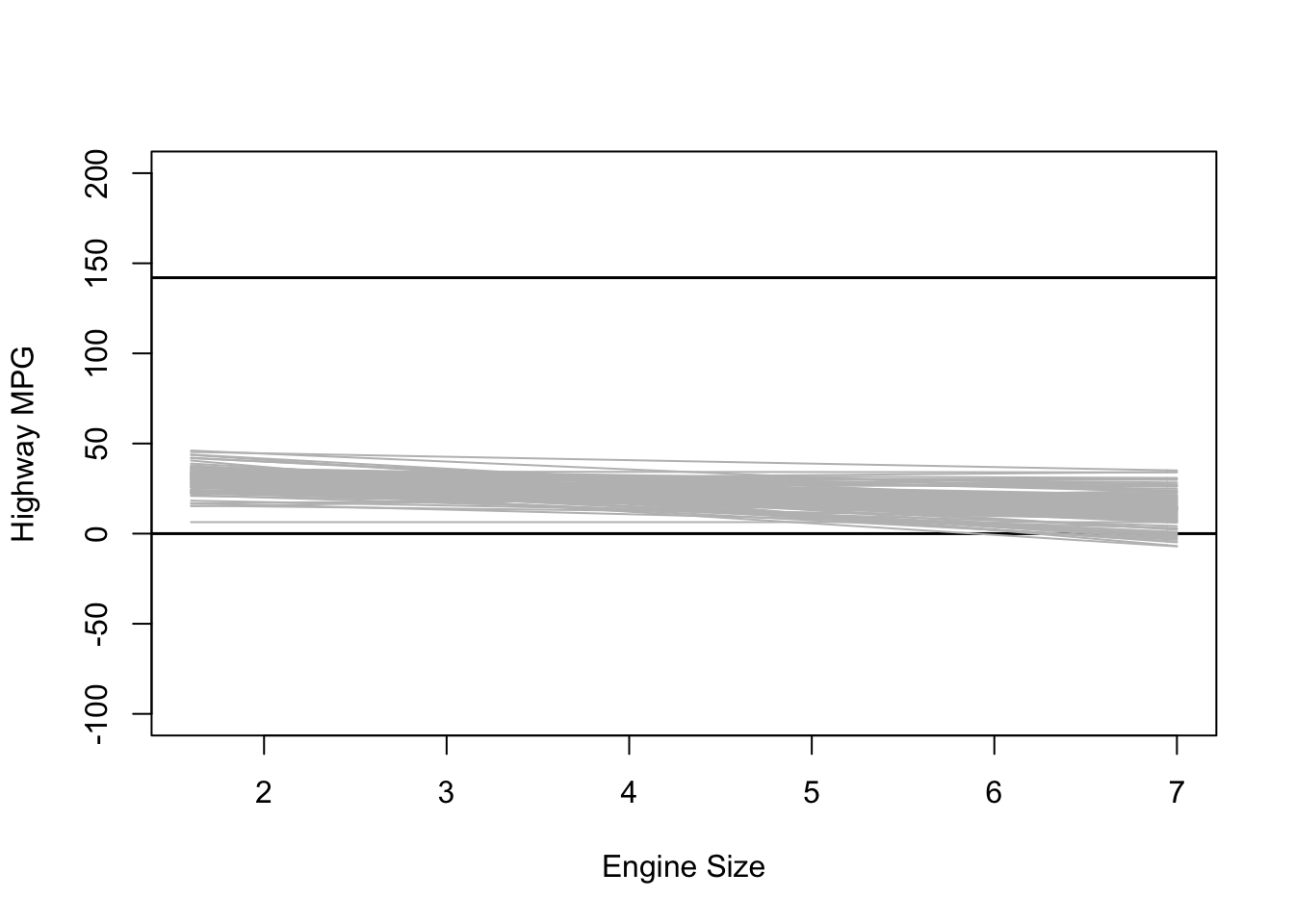# Model Fitting

Now that we know what priors we want to use, we’re ready to fit the model. We’ll estimate three different models each using either “frequentist” priors, weakly informative priors, and informative priors. As a baseline, we’ll estimate the frequentist model.

# First lets create a mean centered version of displ
mpg <-
mpg %>%
mutate(displ_c = displ - mean(displ))

fq.fit <- glm(hwy ~ displ_c,
family = gaussian(),
data = mpg)
summary(fq.fit)
##
## Call:
## glm(formula = hwy ~ displ_c, family = gaussian(), data = mpg)
##
## Deviance Residuals:
##     Min       1Q   Median       3Q      Max
## -7.1039  -2.1646  -0.2242   2.0589  15.0105
##
## Coefficients:
##             Estimate Std. Error t value Pr(>|t|)
## (Intercept)  23.4402     0.2508   93.47   <2e-16 ***
## displ_c      -3.5306     0.1945  -18.15   <2e-16 ***
## ---
## Signif. codes:  0 '***' 0.001 '**' 0.01 '*' 0.05 '.' 0.1 ' ' 1
##
## (Dispersion parameter for gaussian family taken to be 14.71478)
##
##     Null deviance: 8261.7  on 233  degrees of freedom
## Residual deviance: 3413.8  on 232  degrees of freedom
## AIC: 1297.2
##
## Number of Fisher Scoring iterations: 2

## “Frequentist” Prior Model

bfit.1 <- brm(hwy ~ displ_c, # model formula
family = gaussian(), # the likelihood function
data = mpg, # the data the model is using
prior = c(prior(uniform(-10000000, 10000000), class = "Intercept"),
prior(uniform(-10000000, 10000000), class = "b")),
silent = 2,
refresh = 0)
## Warning: It appears as if you have specified a lower bounded prior on a parameter that has no natural lower bound.
## If this is really what you want, please specify argument 'lb' of 'set_prior' appropriately.
## Warning occurred for prior
## b ~ uniform(-1e+07, 1e+07)
## Warning: It appears as if you have specified an upper bounded prior on a parameter that has no natural upper bound.
## If this is really what you want, please specify argument 'ub' of 'set_prior' appropriately.
## Warning occurred for prior
## b ~ uniform(-1e+07, 1e+07)
bfit.1
##  Family: gaussian
##   Links: mu = identity; sigma = identity
## Formula: hwy ~ displ_c
##    Data: mpg (Number of observations: 234)
##   Draws: 4 chains, each with iter = 2000; warmup = 1000; thin = 1;
##          total post-warmup draws = 4000
##
## Population-Level Effects:
##           Estimate Est.Error l-95% CI u-95% CI Rhat Bulk_ESS Tail_ESS
## Intercept    23.44      0.25    22.93    23.92 1.00     3618     2681
## displ_c      -3.53      0.20    -3.91    -3.14 1.00     3977     3043
##
## Family Specific Parameters:
##       Estimate Est.Error l-95% CI u-95% CI Rhat Bulk_ESS Tail_ESS
## sigma     3.86      0.18     3.51     4.24 1.00     4212     3122
##
## Draws were sampled using sampling(NUTS). For each parameter, Bulk_ESS
## and Tail_ESS are effective sample size measures, and Rhat is the potential
## scale reduction factor on split chains (at convergence, Rhat = 1).

## Weakly Informative Prior Model

bfit.2 <- brm(hwy ~ displ_c, # model formula
family = gaussian(), # the likelihood function
data = mpg, # the data the model is using
prior = c(prior(normal(20, 20), class = "Intercept"),
prior(normal(-5, 20), class = "b")),
silent = 2,
refresh = 0)
bfit.2
##  Family: gaussian
##   Links: mu = identity; sigma = identity
## Formula: hwy ~ displ_c
##    Data: mpg (Number of observations: 234)
##   Draws: 4 chains, each with iter = 2000; warmup = 1000; thin = 1;
##          total post-warmup draws = 4000
##
## Population-Level Effects:
##           Estimate Est.Error l-95% CI u-95% CI Rhat Bulk_ESS Tail_ESS
## Intercept    23.44      0.24    22.97    23.91 1.00     4227     3108
## displ_c      -3.53      0.20    -3.92    -3.14 1.00     4033     2920
##
## Family Specific Parameters:
##       Estimate Est.Error l-95% CI u-95% CI Rhat Bulk_ESS Tail_ESS
## sigma     3.86      0.18     3.52     4.23 1.00     4340     3160
##
## Draws were sampled using sampling(NUTS). For each parameter, Bulk_ESS
## and Tail_ESS are effective sample size measures, and Rhat is the potential
## scale reduction factor on split chains (at convergence, Rhat = 1).

## Informative Prior Model

bfit.3 <- brm(hwy ~ displ_c, # model formula
family = gaussian(), # the likelihood function
data = mpg, # the data the model is using
prior = c(prior(normal(25, 5), class = "Intercept"),
prior(normal(-3, 2), class = "b")),
silent = 2,
refresh = 0)
bfit.3
##  Family: gaussian
##   Links: mu = identity; sigma = identity
## Formula: hwy ~ displ_c
##    Data: mpg (Number of observations: 234)
##   Draws: 4 chains, each with iter = 2000; warmup = 1000; thin = 1;
##          total post-warmup draws = 4000
##
## Population-Level Effects:
##           Estimate Est.Error l-95% CI u-95% CI Rhat Bulk_ESS Tail_ESS
## Intercept    23.44      0.26    22.92    23.95 1.00     3935     3135
## displ_c      -3.53      0.20    -3.91    -3.13 1.00     3840     2804
##
## Family Specific Parameters:
##       Estimate Est.Error l-95% CI u-95% CI Rhat Bulk_ESS Tail_ESS
## sigma     3.86      0.18     3.52     4.24 1.00     3659     3011
##
## Draws were sampled using sampling(NUTS). For each parameter, Bulk_ESS
## and Tail_ESS are effective sample size measures, and Rhat is the potential
## scale reduction factor on split chains (at convergence, Rhat = 1).

# Convergence Diagnostics

We always need to check the convergence of each model because they are estimated using MCMC. There are a couple ways we can check convergence. The first way is to check convergence is with a visual inspection of the traceplots. The bayesplot package makes it easy to make traceplots.

library(bayesplot)
## This is bayesplot version 1.8.1
## - Online documentation and vignettes at mc-stan.org/bayesplot
## - bayesplot theme set to bayesplot::theme_default()
##    * Does _not_ affect other ggplot2 plots
##    * See ?bayesplot_theme_set for details on theme setting
mcmc_trace(bfit.2)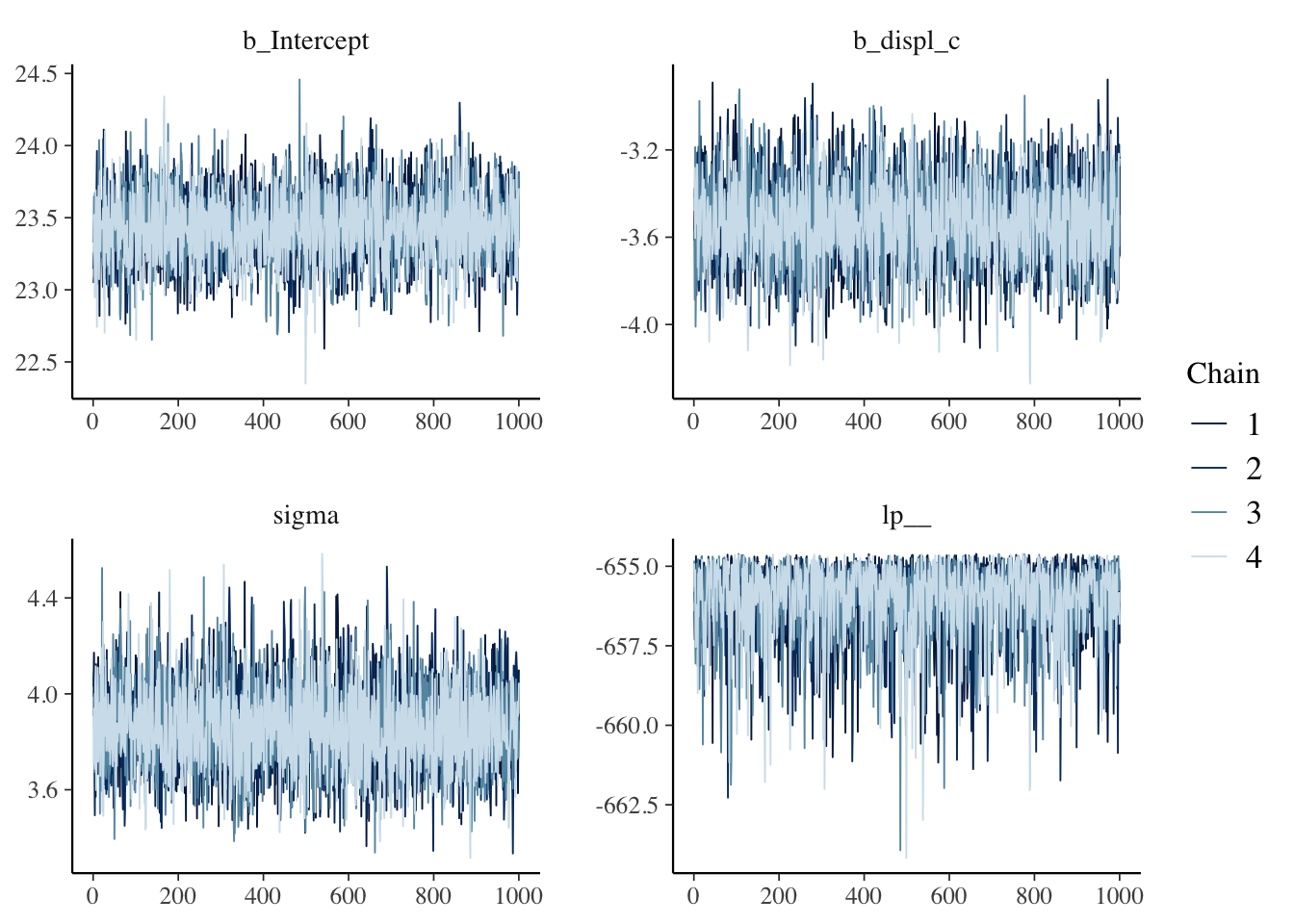The “fuzzy caterpillar” is what we want to see. Each MCMC chain is a separate line and we want to see each line “mixing” with one another. If they will all separate that would mean that each chain has a different estimate.

Another way to check the model’s convergence is to look at the Rhat values.

bfit.2
##  Family: gaussian
##   Links: mu = identity; sigma = identity
## Formula: hwy ~ displ_c
##    Data: mpg (Number of observations: 234)
##   Draws: 4 chains, each with iter = 2000; warmup = 1000; thin = 1;
##          total post-warmup draws = 4000
##
## Population-Level Effects:
##           Estimate Est.Error l-95% CI u-95% CI Rhat Bulk_ESS Tail_ESS
## Intercept    23.44      0.24    22.97    23.91 1.00     4227     3108
## displ_c      -3.53      0.20    -3.92    -3.14 1.00     4033     2920
##
## Family Specific Parameters:
##       Estimate Est.Error l-95% CI u-95% CI Rhat Bulk_ESS Tail_ESS
## sigma     3.86      0.18     3.52     4.23 1.00     4340     3160
##
## Draws were sampled using sampling(NUTS). For each parameter, Bulk_ESS
## and Tail_ESS are effective sample size measures, and Rhat is the potential
## scale reduction factor on split chains (at convergence, Rhat = 1).
# rhat plot
rhats <- rhat(bfit.2)
mcmc_rhat(rhats) + xlim(1, 1.001)
## Scale for 'x' is already present. Adding another scale for 'x', which will
## replace the existing scale.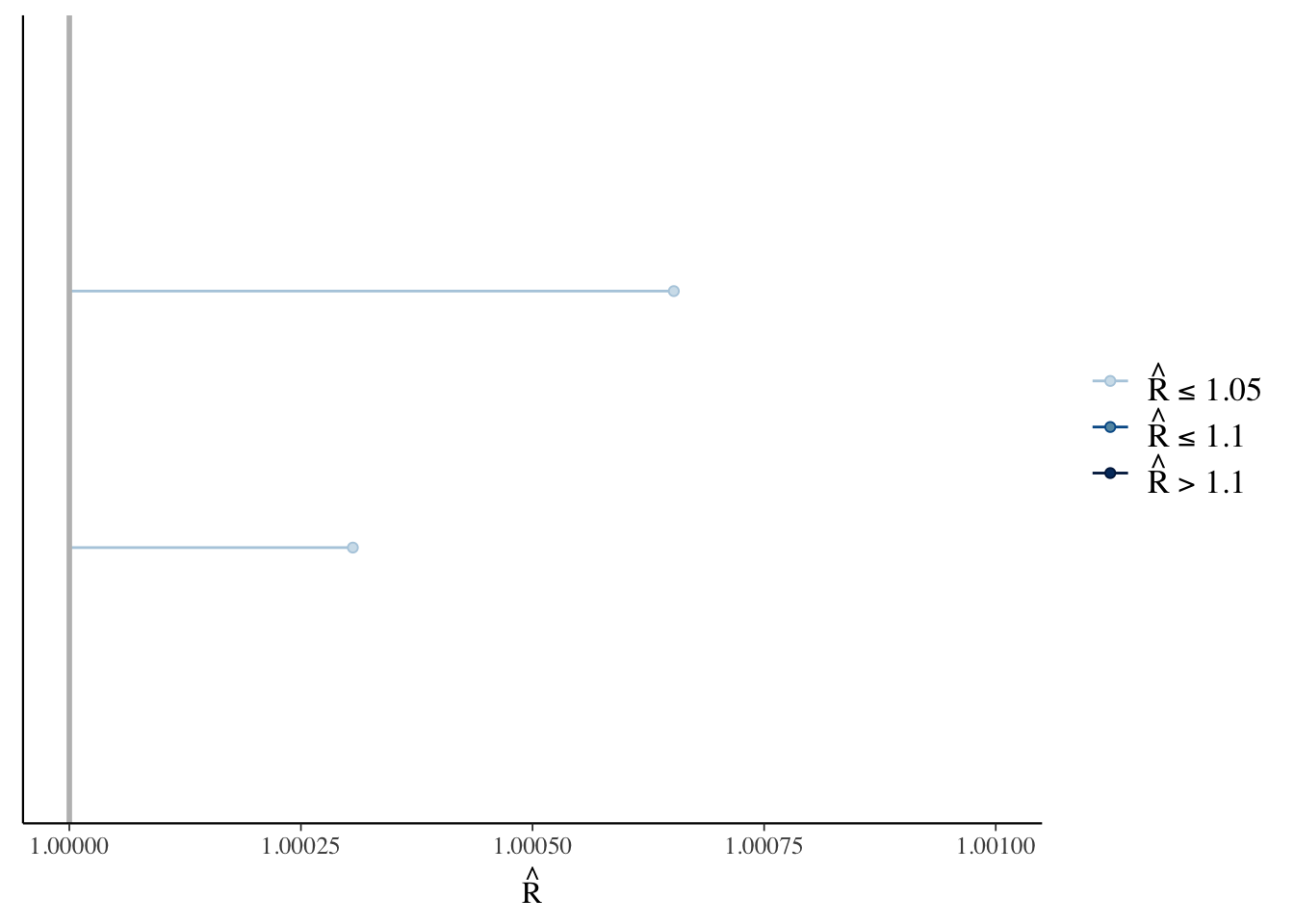# Posterior Predictive Checks

One of the unique things about Bayesian analysis is the fact that we can preform posterior predictive checks. What is a posterior predictive check? The basic idea is that if we can create a model that accurately predicts the data we have, then we should be able to use the same model to generate new data points.

pp_check(bfit.2, type = "dens_overlay", ndraws = 100)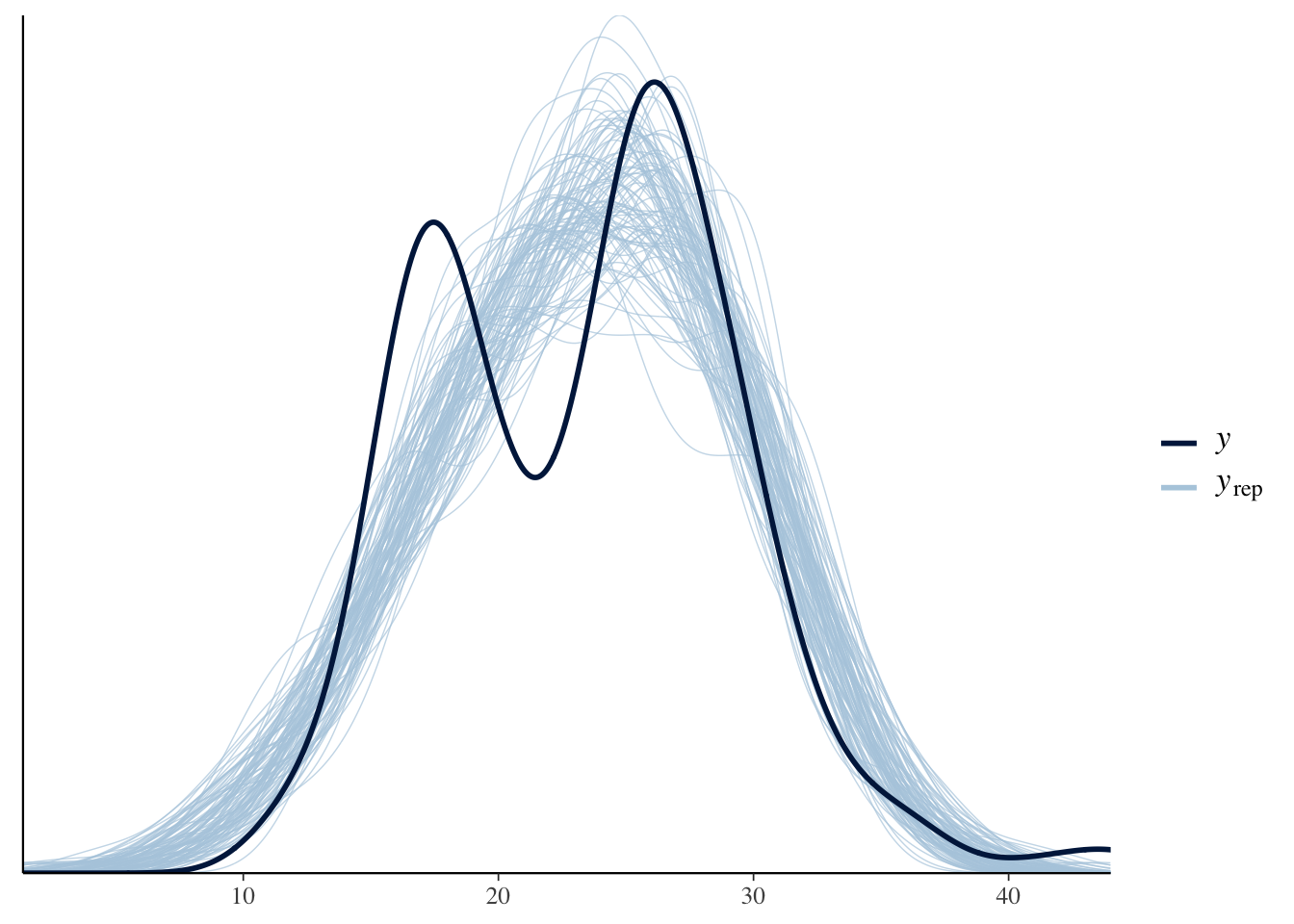$$y$$ is the actual data and $$y_{rep}$$ is the data that was generated from the model. An accurate model would be able to generate new data points that follow the same distribution as the actual data. Based on this plot, it looks like we are missing some of the variation in the middle.

# Results Table

library(broom.mixed)
library(knitr)
library(kableExtra)
##
## Attaching package: 'kableExtra'
## The following object is masked from 'package:dplyr':
##
##     group_rows
results.table <-
tidyMCMC(bfit.3,
robust = TRUE,
conf.int = TRUE,
conf.method = "HPDinterval") %>%
mutate(conf.int = sprintf("(%.1f, %.1f)", conf.low, conf.high)) %>%
select(term, estimate, std.error, conf.int)

kable(results.table,
escape = FALSE,
booktabs = TRUE,
digits = 2,
align = c("l", "r", "r", "c"),
col.names = c("Parameter", "Point Estimate", "SD",
"95% HDI Interval ")) %>%
kable_styling(latex_options = c("striped", "hold_position"))
Parameter Point Estimate SD 95% HDI Interval
b_Intercept 23.44 0.26 (23.0, 24.0)
b_displ_c -3.53 0.20 (-3.9, -3.1)
sigma 3.86 0.17 (3.5, 4.2)

# Results Visualization

Just like in frequentist statistics, we can create a coefficient plot where we see the point estimate and the 95% interval.

mcmc_plot(bfit.2, variable = "b_displ_c")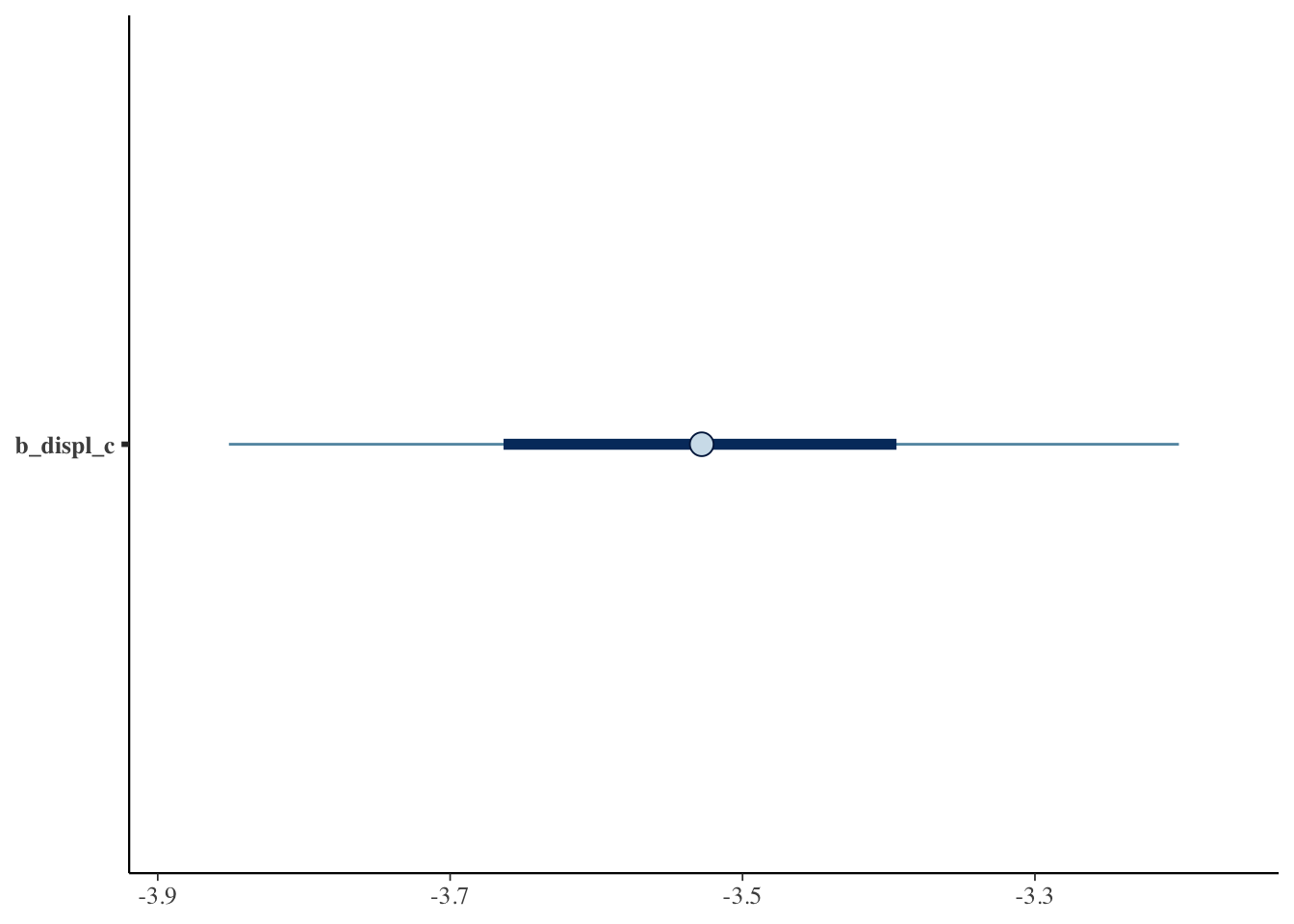One of the best things about Bayesian analysis, however, is that we don’t just get a single point estimate. Instead we get an entire distribution of estimates. This is distribution is called the posterior distribution. We can plot it like a histogram.

mcmc_hist(bfit.2, pars = "b_displ_c")
## stat_bin() using bins = 30. Pick better value with binwidth.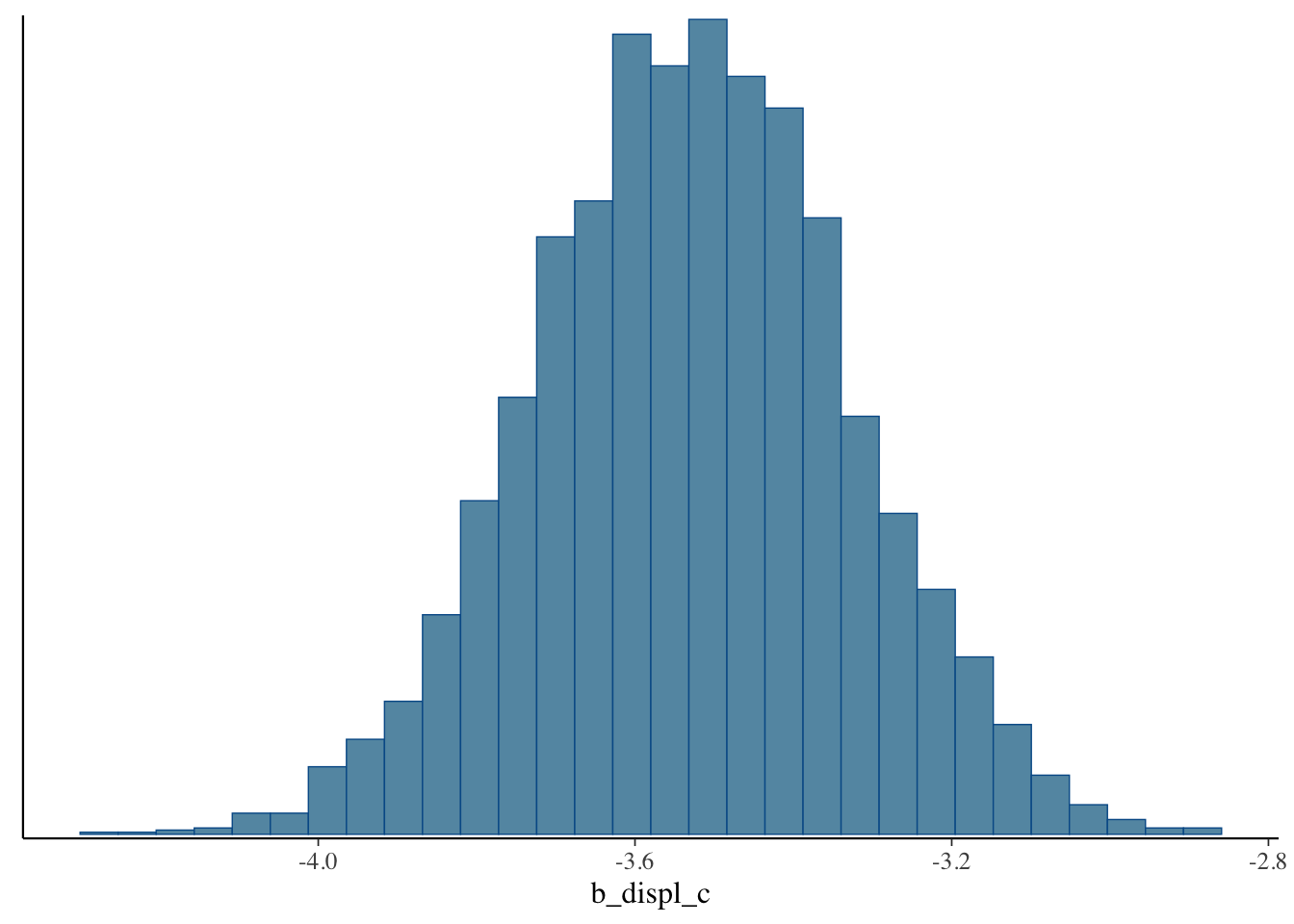mcmc_areas(bfit.2, pars = "b_displ_c", prob = 0.95)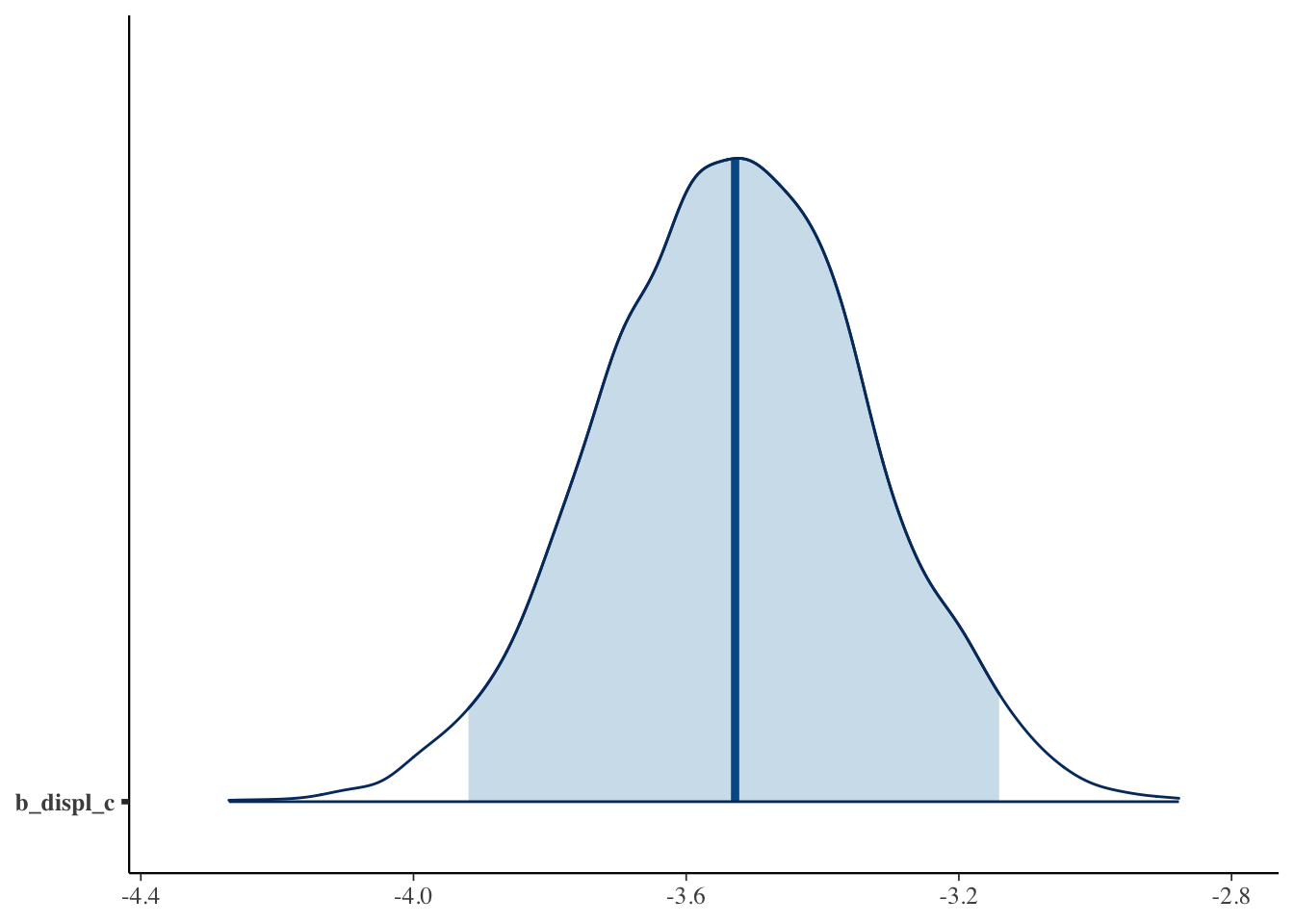# Results Interpretation

The interpretation of the results is very easy. Let’s pull them up:

bfit.2
##  Family: gaussian
##   Links: mu = identity; sigma = identity
## Formula: hwy ~ displ_c
##    Data: mpg (Number of observations: 234)
##   Draws: 4 chains, each with iter = 2000; warmup = 1000; thin = 1;
##          total post-warmup draws = 4000
##
## Population-Level Effects:
##           Estimate Est.Error l-95% CI u-95% CI Rhat Bulk_ESS Tail_ESS
## Intercept    23.44      0.24    22.97    23.91 1.00     4227     3108
## displ_c      -3.53      0.20    -3.92    -3.14 1.00     4033     2920
##
## Family Specific Parameters:
##       Estimate Est.Error l-95% CI u-95% CI Rhat Bulk_ESS Tail_ESS
## sigma     3.86      0.18     3.52     4.23 1.00     4340     3160
##
## Draws were sampled using sampling(NUTS). For each parameter, Bulk_ESS
## and Tail_ESS are effective sample size measures, and Rhat is the potential
## scale reduction factor on split chains (at convergence, Rhat = 1).

By default, brms shows us the mean point estimate but we could just as easily use the median or mode.

• The mean point estimate is shown in the Estimate column.

• Est.Error is the standard deviation of the posterior distribution.

• l-95% CI and u-95% CI are the lower and upper 95% credible interval estimates. Remember, the 95% credible interval tells us the range of values that will contain the true value with a 95% probability.

• Rhat tells us about convergence. A value greater than 1.1 is bad.

• Bulk_ESS and Tail_ESS show the number of independent draws. The higher the better.

Let’s interpret the effect of displ_c on hwy: A one unit change in displ_c is associated with a $$-3.53$$ decrease in a vehicle’s highway MPG. This effect has a standard deviation of $$0.19$$ and there is a 95% probability that the true value is between $$-3.90$$ and $$-3.14$$.

# Bonus: How Influential Are Our Priors?

To see how much our choice of priors influenced the posterior distribution we just compare the two distributions. To do this, we can draw samples from our priors when we estimate the model using the argument sample_prior = "yes". This will save the prior distributions so we can plot them later.

bfit.2 <- brm(hwy ~ displ_c, # model formula
family = gaussian(), # the likelihood function
data = mpg, # the data the model is using
prior = c(prior(normal(20, 20), class = "Intercept"),
prior(normal(-5, 20), class = "b")),
silent = 2,
refresh = 0,
sample_prior = "yes")

priors <- prior_draws(bfit.2)
head(priors)
##    Intercept          b     sigma
## 1  27.926016 -53.173939  2.927436
## 2  33.417121  -8.934286  4.826794
## 3   7.003081  -7.465716  6.218460
## 4  14.252230  15.807468  8.453561
## 5  28.373259  14.919524 16.521201
## 6 -25.861000  -2.497979 10.068648

This shows all 4,000 draws from the priors that we used. If we summarize them, we’ll see that the mean is just about equal to what we set it to.

summary(priors)
##    Intercept             b               sigma
##  Min.   :-49.913   Min.   :-70.105   Min.   :  0.00008
##  1st Qu.:  6.766   1st Qu.:-18.831   1st Qu.:  2.67372
##  Median : 20.292   Median : -5.710   Median :  5.88203
##  Mean   : 20.202   Mean   : -5.543   Mean   :  8.29821
##  3rd Qu.: 33.768   3rd Qu.:  7.688   3rd Qu.: 10.61485
##  Max.   : 90.531   Max.   : 65.585   Max.   :234.76037

Now we can plot them:

ggplot() +
geom_density(data = priors, aes(x = b)) +
labs(title = "Prior Distribution") +
theme_classic()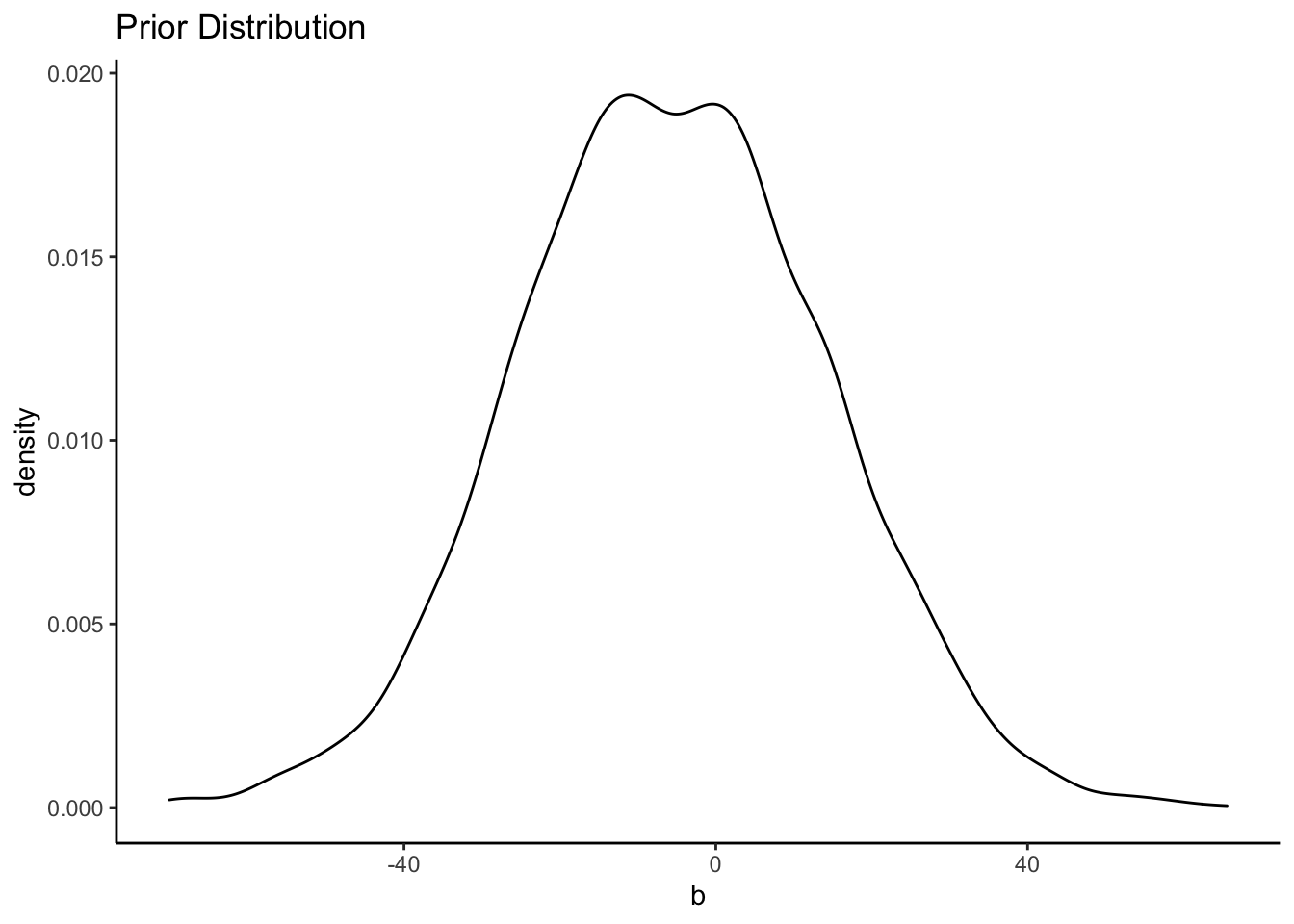We can also plot the posterior distribution:

posteriors <- as.data.frame(bfit.2)

ggplot() +
geom_density(data = posteriors, aes(x = b_displ_c), color = "blue") +
labs(title = "Posterior Distribution") +
theme_classic()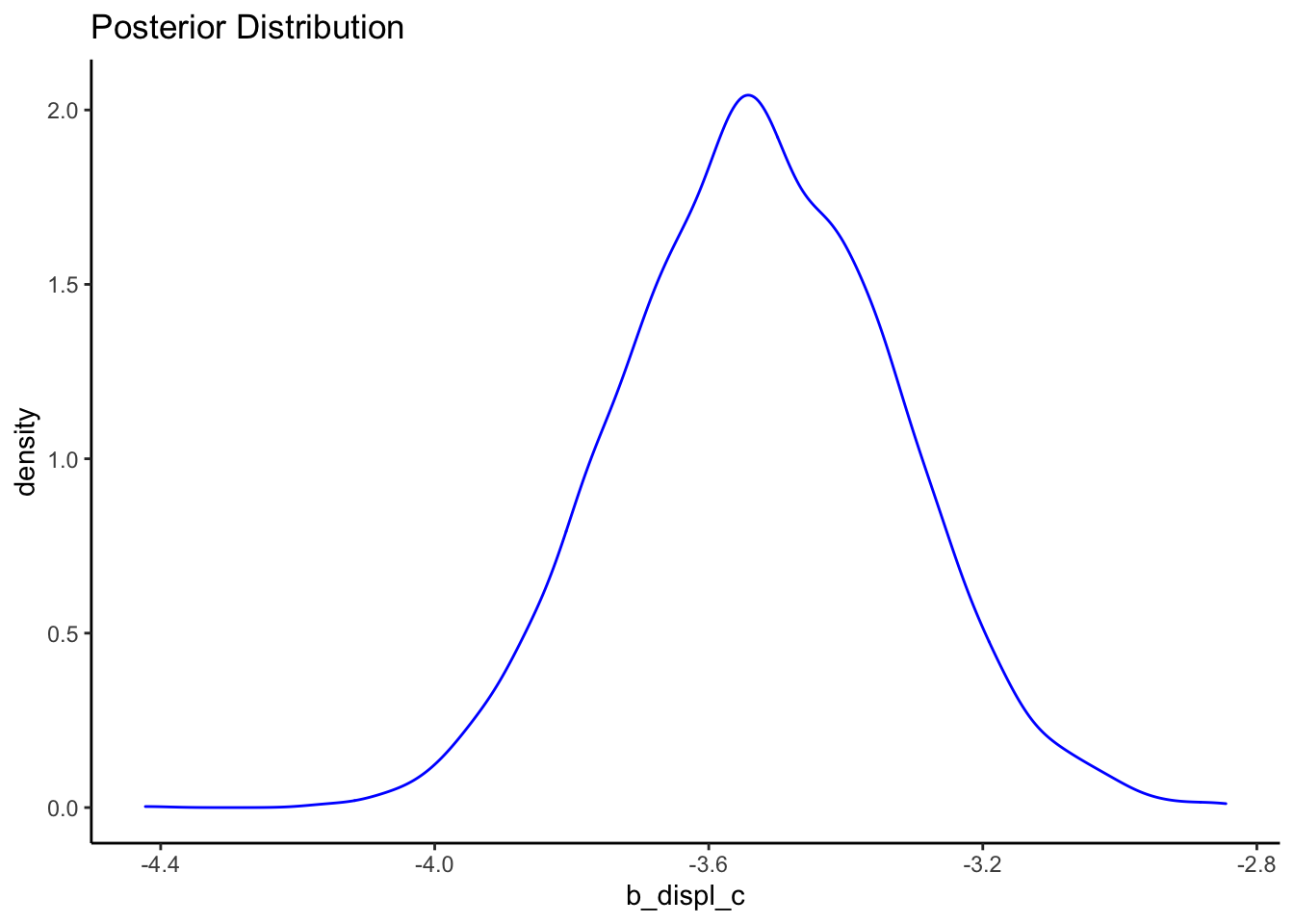It’s easy to see the comparison of the prior distribution to the posterior if we put them on the same plot. Since the x-axes are so different, however, we’ll need to make some manual adjustments.

ggplot() +
geom_density(data = priors, aes(x = b)) +
geom_density(data = posteriors, aes(x = b_displ_c), color = "blue") +
labs(title = "Prior Distribution vs. Posterior Distribution") +
xlim(-10, 10) +
theme_classic()
## Warning: Removed 2542 rows containing non-finite values (stat_density).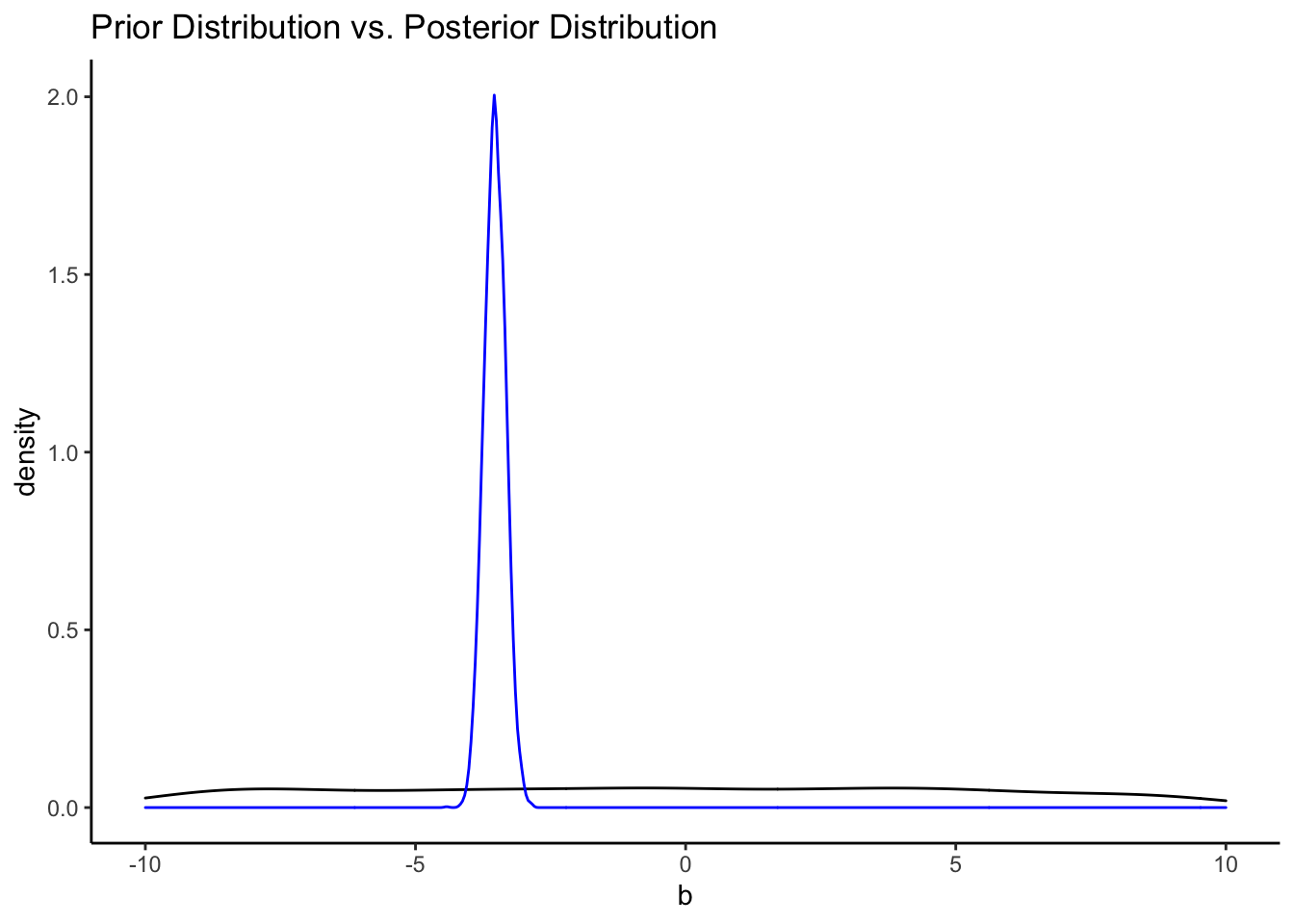That’s a pretty uninformative prior. How do the priors from the informative model compare?

bfit.3 <- brm(hwy ~ displ_c, # model formula
family = gaussian(), # the likelihood function
data = mpg, # the data the model is using
prior = c(prior(normal(25, 5), class = "Intercept"),
prior(normal(-3, 2), class = "b")),
silent = 2,
refresh = 0,
sample_prior = "yes")

priors <- prior_draws(bfit.3)
posteriors <- as.data.frame(bfit.3)

ggplot() +
geom_density(data = priors, aes(x = b)) +
geom_density(data = posteriors, aes(x = b_displ_c), color = "blue") +
labs(title = "Prior Distribution vs. Posterior Distribution") +
xlim(-10, 10) +
theme_classic()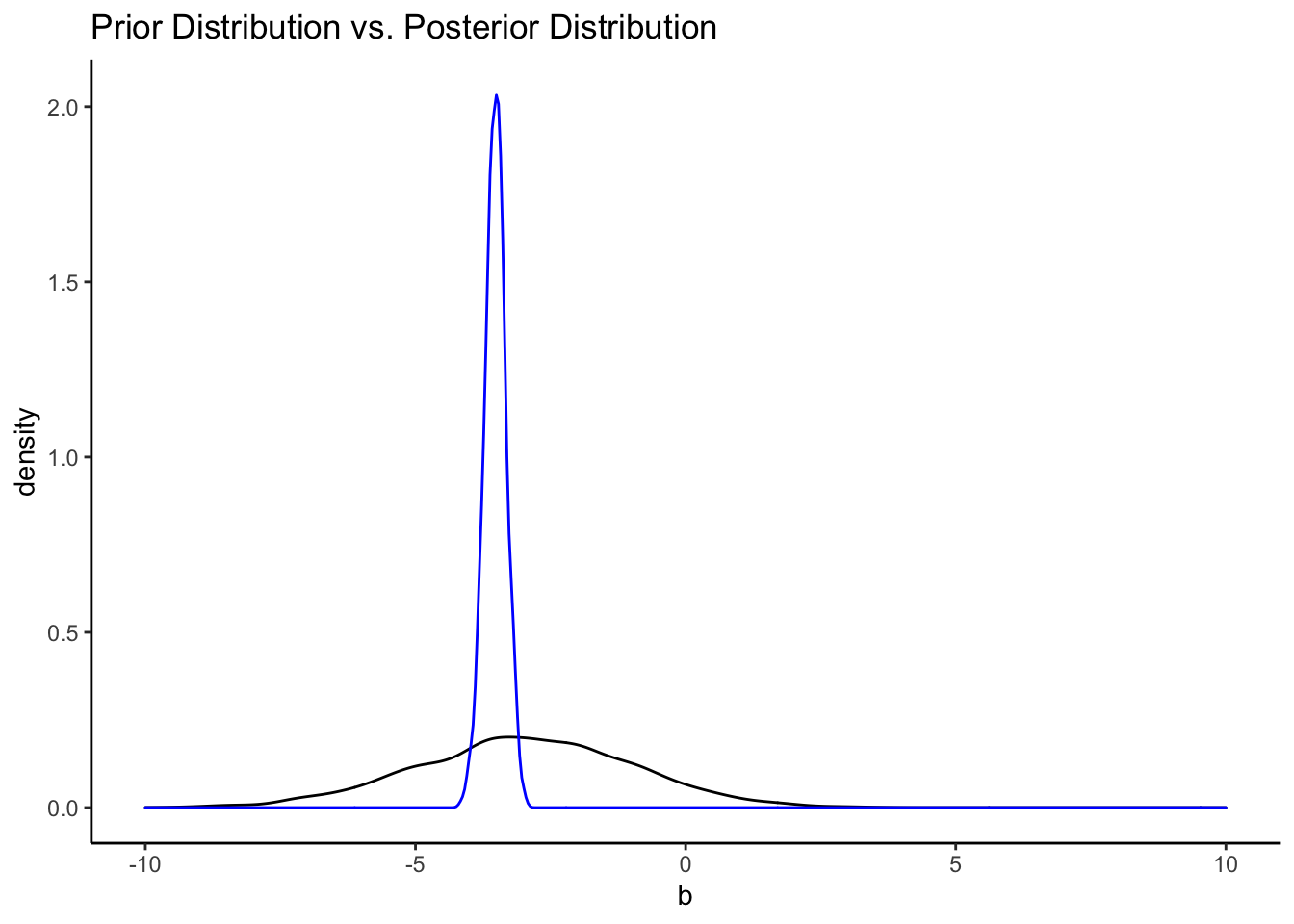# Resources